# A Guide to U.S. Presidential Elections

Click on any date below to see the results for that year's election.

 1789 1868 1948 1792 1872 1952 1796 1876 1956 1800 1880 1960 1804 1884 1964 1808 1888 1968 1812 1892 1972 1816 1896 1976 1820 1900 1980 1824 1904 1984 1828 1908 1988 1832 1912 1992 1836 1916 1996 1840 1920 2000 1844 1924 2004 1848 1928 2008 1852 1932 2012 1856 1936 2016 1860 1940 1864 1944

Or view an Excel spreadsheet containing the electoral vote count, state by state (requires a zip/unzip utility).

## 1789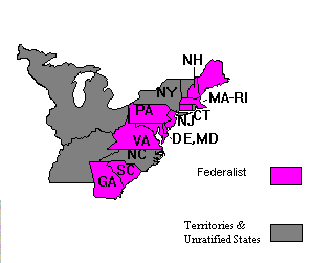Electoral Vote:

Federalist (George Washington) = 69.

New York did not have its electors ready in time, while North Carolina and Rhode Island had not yet approved the Constitution, so they were inelligible to vote.

## 1792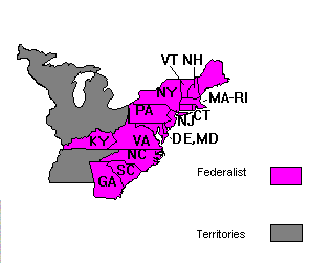Electoral Vote:

Federalist (George Washington) = 132.

## 1796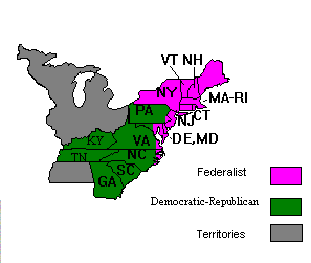Electoral Vote:

Federalist (John Adams) = 71.
Democratic-Republican (Thomas Jefferson) = 68.
Total = 139.

## 1800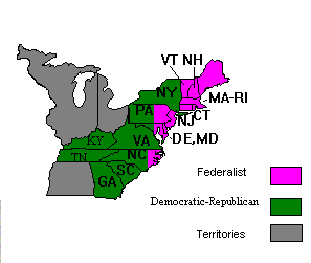Electoral Vote:

Democratic-Republican (Thomas Jefferson) = 73.
Federalist (John Adams) = 65.
Total = 138.

## 1804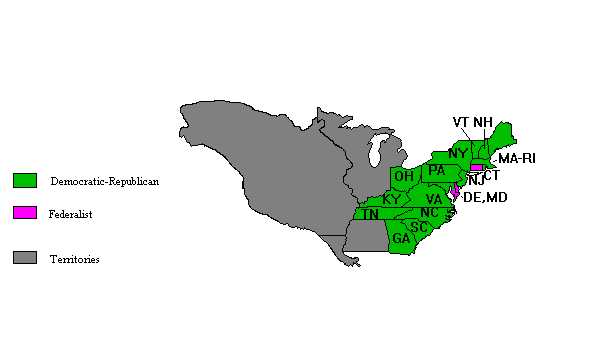Electoral Vote:

Democratic-Republican (Thomas Jefferson) = 162.
Federalist (Charles Pinckney) = 14.
Total = 176.

## 1808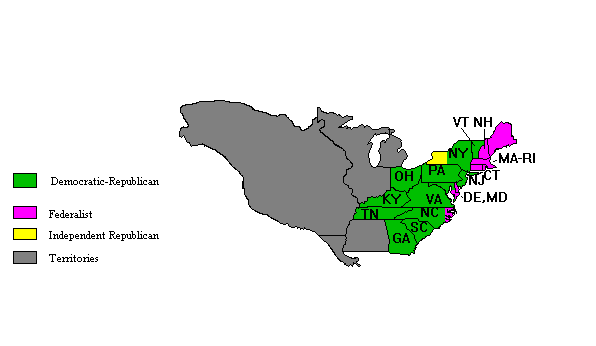Electoral Vote:

Democratic-Republican (James Madison) = 122.
Federalist (Charles Pinckney) = 47.
Independent Republican (George Clinton) = 6.
Not voting = 1.
Total = 176.

## 1812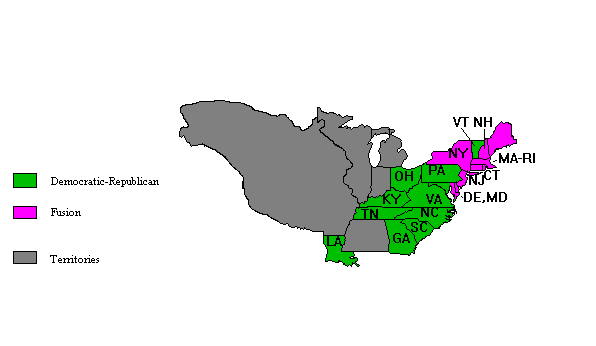Electoral Vote:

Democratic-Republican (James Madison) = 128.
Fusion (De Witt Clinton) = 89.
Not voting = 1.
Total = 218.

Note: Though nominated by the Federalists, Clinton was a Republican, and enjoyed the support of Republicans who opposed the War of 1812.

## 1816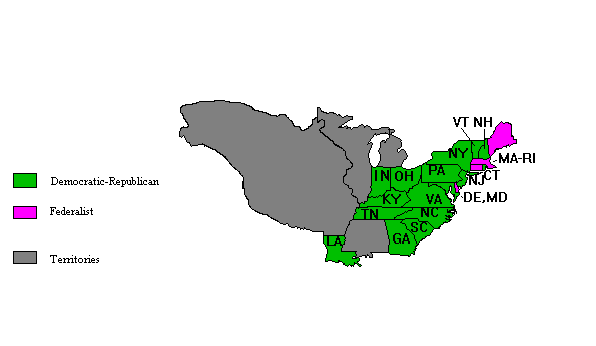Electoral Vote:

Democratic-Republican (James Monroe) = 183.
Federalist (Rufus King) = 34.
Not voting = 4.
Total = 221.

## 1820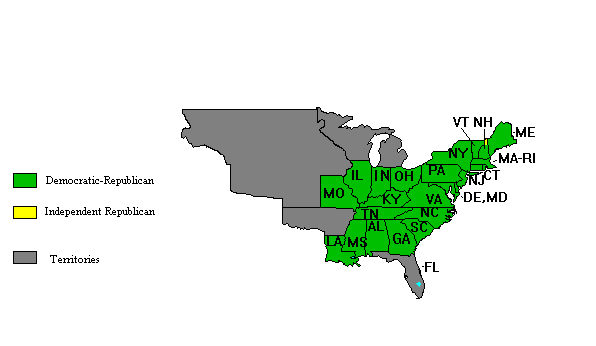Electoral Vote:

Democratic-Republican (James Monroe) = 231.
Independent Republican (John Quincy Adams) = 1.
Not voting = 3.
Total = 221.

## 1824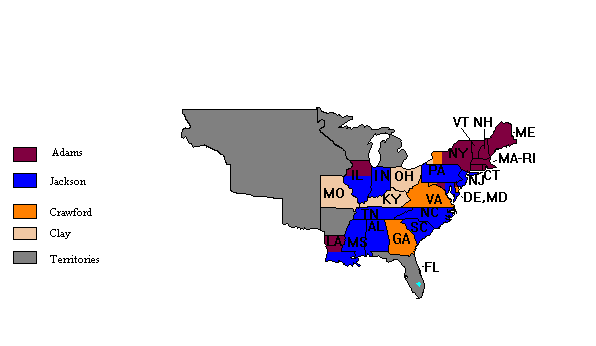All candidates were Democratic-Republicans, so no party was a factor in this election. None of them got a majority of the vote (both popular and electoral), so the election was thrown to the House of Representatives, which chose John Quincy Adams as the winner.

Electoral Vote:

Andrew Jackson = 99.
John Quincy Adams = 84.
William Crawford = 41.
Henry Clay = 37.
Total = 261.

Popular Vote:

Andrew Jackson = 153,544 (43%).
John Quincy Adams = 108,740 (31%).
Henry Clay = 47,136 (13%).
William Crawford = 46,618 (13%).
Total = 356,038.

## 1828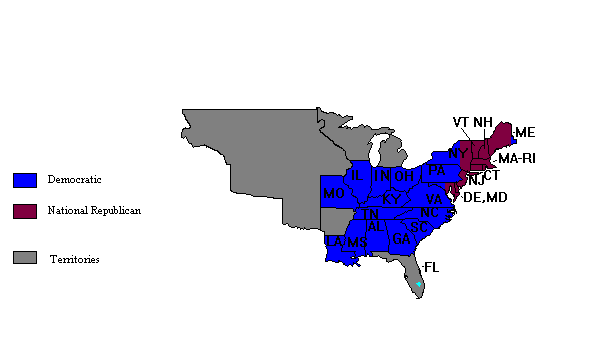Electoral Vote:

Democratic (Andrew Jackson) = 178.
National Republican (John Quincy Adams) = 83.
Total = 261.

Popular Vote:

Democratic (Andrew Jackson) = 647,286 (56%).
National Republican (John Quincy Adams) = 508,064 (44%).
Total = 1,155,350.

## 1832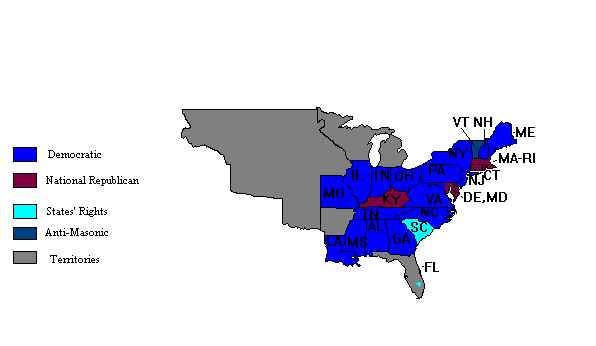Electoral Vote:

Democratic (Andrew Jackson) = 219.
National Republican (Henry Clay) = 49.
States' Right (John Floyd) = 11.
Anti-Masonic (William Wirt) = 7.
Not voting = 2.
Total = 288.

Popular Vote:

Democratic (Andrew Jackson) = 687,502 (55%).
National Republican (Henry Clay) = 530,189 (42.4%).
Anti-Masonic (William Wirt) = 33,108 (2.6%).
Total = 1,250,799.

## 1836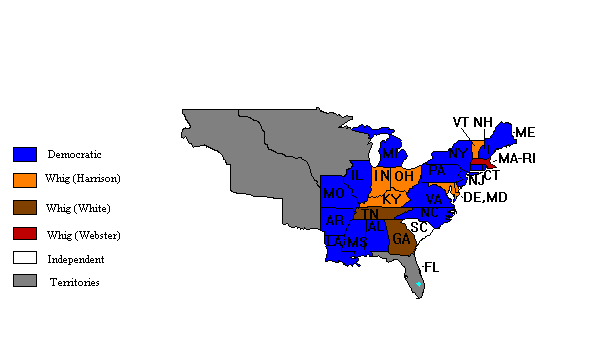Electoral Vote:

Democratic (Martin Van Buren) = 170.
Whig (William Henry Harrison) = 73.
Whig (Hugh Lawson White) = 26.
Whig (Daniel Webster) = 14.
Independent (Willie P. Mangum) = 11.
Total = 294.

Popular Vote:

Democratic (Martin Van Buren) = 764,176 (51%).
Whig (William Henry Harrison) = 550,816 (36.5%).
Whig (Hugh Lawson White) = 146,107 (10%).
Whig (Daniel Webster) = 41,201 (2.5%).
Total = 1,502,300.

## 1840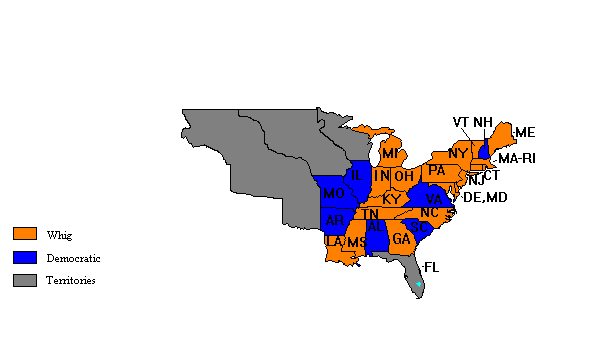Electoral Vote:

Whig (William Henry Harrison) = 234.
Democratic (Martin Van Buren) = 60.
Total = 294.

Popular Vote:

Whig (William Henry Harrison) = 1,250,390 (52.4%).
Democratic (Martin Van Buren) = 1,128,854 (47.3%).
Liberty (James G. Birney) = 7,069 (0.3%).
Total = 2,386,313.

## 1844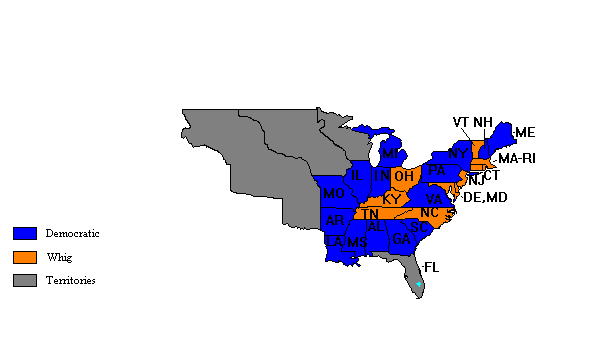Electoral Vote:

Democratic (James K. Polk) = 170.
Whig (Henry Clay) = 105.
Total = 275.

Popular Vote:

Democratic (James K. Polk) = 1,337,243 (49.6%).
Whig (Henry Clay) = 1,299,062 (48.1%).
Liberty (James G. Birney) = 62,300 (2.3%).
Total = 2,698,605.

## 1848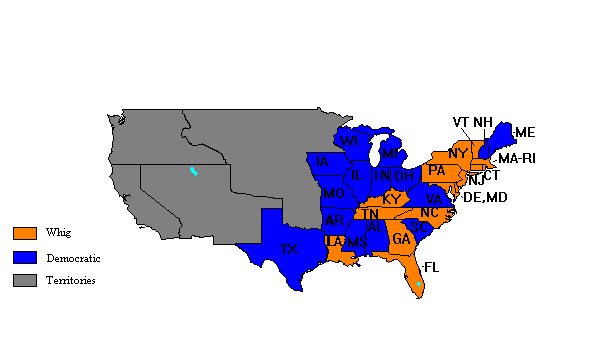Electoral Vote:

Whig (Zachary Taylor) = 163.
Democratic (Lewis Cass) = 127.
Total = 290.

Popular Vote:

Whig (Zachary Taylor) = 1,360,099 (47.5%).
Democratic (Lewis Cass) = 1,220,544 (42.5%).
Free Soil (Martin Van Buren) = 291,263 (10%).
Total = 2,871,906.

## 1852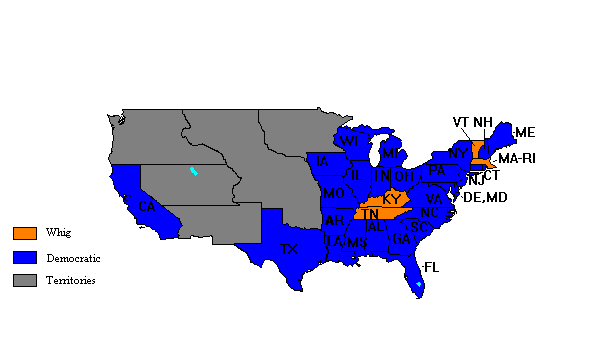Electoral Vote:

Democratic (Franklin Pierce) = 254.
Whig (Winfield Scott) = 42.
Total = 296.

Popular Vote:

Democratic (Franklin Pierce) = 1,601,274 (51%).
Whig (Winfield Scott) = 1,386,580 (44%).
Free Soil (John Parker Hale) = 155,825 (5%).
Total = 3,143,679.

## 1856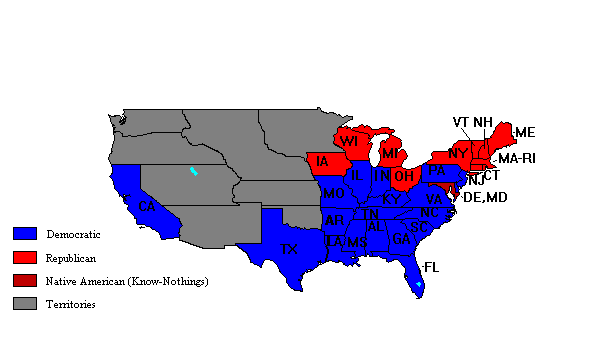Electoral Vote:

Democratic (James Buchanan) = 174.
Republican (John C. Fr�mont) = 114.
Native American/Know-Nothings (Millard Fillmore) = 8.
Total = 296.

Popular Vote:

Democratic (James Buchanan) = 1,838,169 (45.3%).
Republican (John C. Fr�mont) = 1,341,264 (33.1%).
Native American/Know-Nothings (Millard Fillmore) = 874,534 (21.6%).
Total = 4,053,967.

## 1860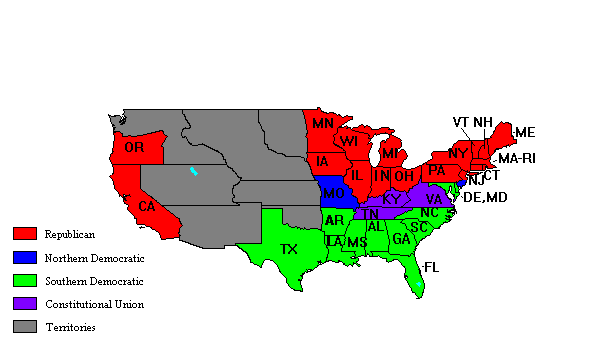Electoral Vote:

Republican (Abraham Lincoln) = 180.
Southern Democratic (John C. Breckinridge) = 72.
Constitutional Union (John Bell) = 39.
Northern Democratic (Stephen A. Douglas) = 12.
Total = 303.

Popular Vote:

Republican (Abraham Lincoln) = 1,865,593 (39.8%).
Northern Democratic (Stephen A. Douglas) = 1,382,713 (29.5%).
Southern Democratic (John C. Breckinridge) = 848,356 (18.1%).
Constitutional Union (John Bell) = 592,906 (12.6%).
Total = 4,689,568.

## 1864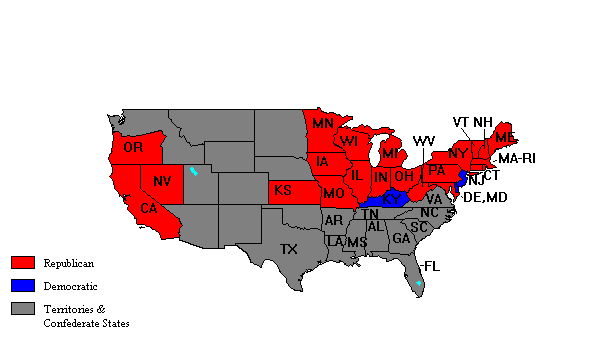Electoral Vote:

Republican (Abraham Lincoln) = 212.
Democratic (George McClellan) = 21.
Not voting = 81.
Total = 314.

Popular Vote:

Republican (Abraham Lincoln) = 2,206,938 (55%).
Democratic (George McClellan) = 1,803,787 (45%).
Total = 4,010,725.

## 1868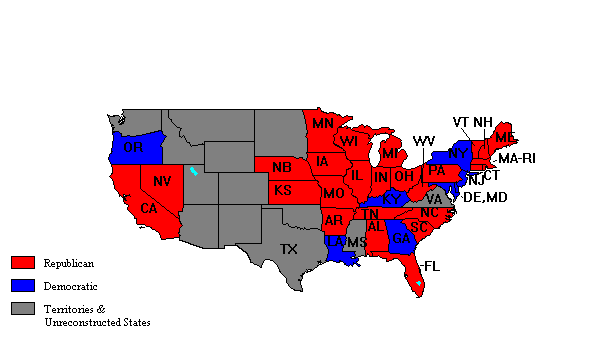Electoral Vote:

Republican (Ulysses S. Grant) = 214.
Democratic (Horatio Seymour) = 80.
Not voting = 23.
Total = 317.

Popular Vote:

Republican (Ulysses S. Grant) = 3,013,421 (52.7%).
Democratic (Horatio Seymour) = 2,706,829 (47.3%).
Total = 5,720,250.

## 1872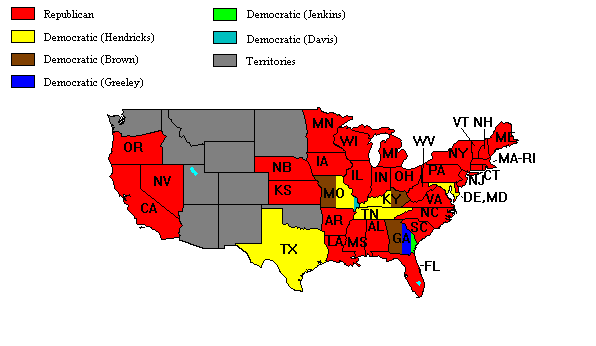Electoral Vote:

Republican (Ulysses S. Grant) = 286.
Democratic (Thomas A. Hendricks) = 42.
Democratic (Benjamin Gratz Brown) = 18.
Democratic (Horace Greeley) = 3.
Democratic (Charles J. Jenkins) = 2.
Democratic (David Davis) = 1.
Not voting = 14.
Total = 366.

Popular Vote:

Republican (Ulysses S. Grant) = 3,597,132 (55.6%).
Democratic (Horace Greeley) = 2,834,125 (43.9%).
Minor candidates = 35,097 (0.5%).
Total = 6,466,354.

## 1876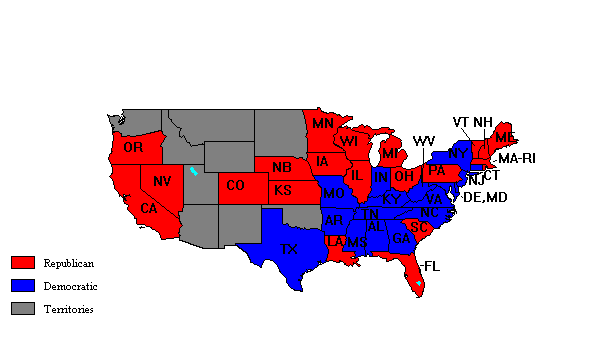Electoral Vote:

Republican (Rutherford B. Hayes) = 185.
Democratic (Samuel J. Tilden) = 184.
Total = 369.

Popular Vote:

Democratic (Samuel J. Tilden) = 4,300,590 (51%).
Republican (Rutherford B. Hayes) = 4,036,298 (47.9%).
Minor candidates = 93,895 (1.1%).
Total = 8,430,783.

## 1880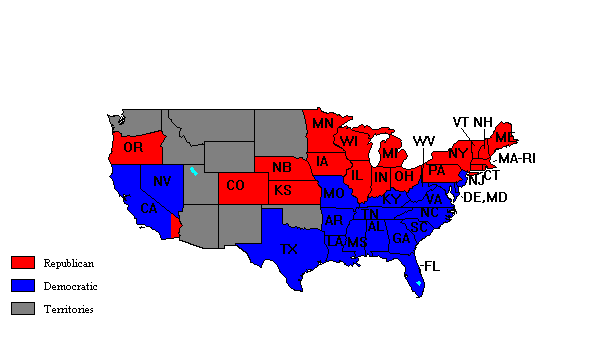Electoral Vote:

Republican (James A. Garfield) = 214.
Democratic (Winfield Scott Hancock) = 155.
Total = 369.

Popular Vote:

Republican (James A. Garfield) = 4,454,416 (48.3%).
Democratic (Winfield Scott Hancock) = 4,444,952 (48.2%).
Minor candidates = 319,583 (3.5%).
Total = 9,218,951.

## 1884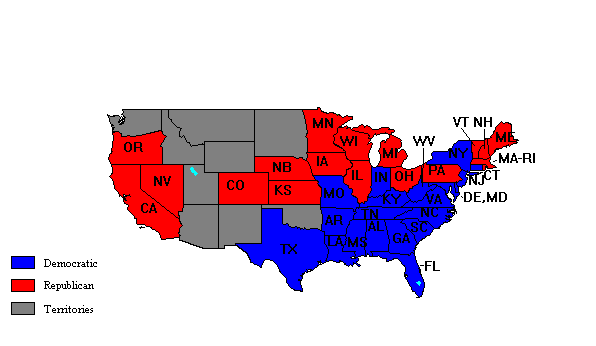Electoral Vote:

Democratic (Grover Cleveland) = 219.
Republican (James G. Blaine) = 182.
Total = 401.

Popular Vote:

Democratic (Grover Cleveland) = 4,874,986 (48.5%).
Republican (James G. Blaine) = 4,851,981 (48.3%).
Minor candidates = 325,739 (3.2%).
Total = 10,052,706.

## 1888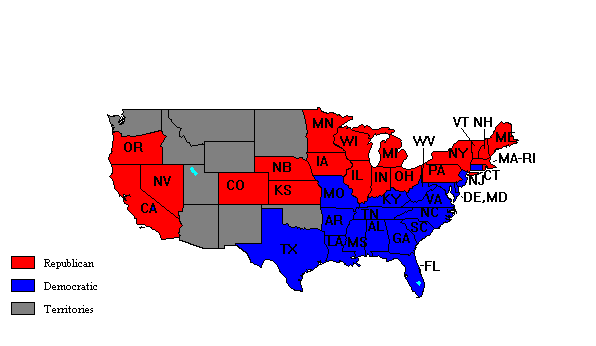Electoral Vote:

Republican (Benjamin Harrison) = 233.
Democratic (Grover Cleveland) = 168.
Total = 401.

Popular Vote:

Democratic (Grover Cleveland) = 5,540,309 (48.7%).
Republican (Benjamin Harrison) = 5,439,853 (47.8%).
Minor candidates = 400,859 (3.5%).
Total = 11,381,021.

## 1892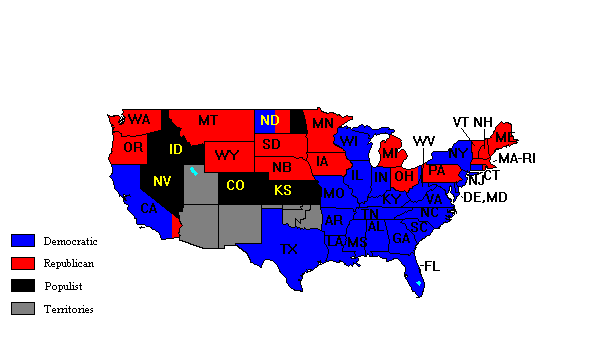Electoral Vote:

Democratic (Grover Cleveland) = 277.
Republican (Benjamin Harrison) = 145.
Populist (James B. Weaver) = 22.
Total = 444.

Popular Vote:

Democratic (Grover Cleveland) = 5,551,883 (46%).
Republican (Benjamin Harrison) = 5,179,244 (43%).
Populist (James B. Weaver) = 1,024,280 (8.5%).
Prohibition (John Bidwell) = 270,770 (2.2%).
Minor candidates = 29,920 (0.3%).
Total = 12,056,097.

## 1896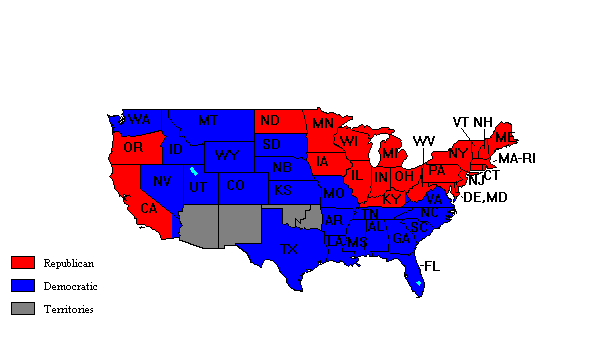Electoral Vote:

Republican (William McKinley) = 271.
Democratic (William Jennings Bryan) = 176.
Total = 447.

Popular Vote:

Republican (William McKinley) = 7,104,779 (51%).
Democratic (William Jennings Bryan) = 6,502,925 (46.7%).
Minor candidates = 315,398 (2.3%).
Total = 13,923,102.

## 1900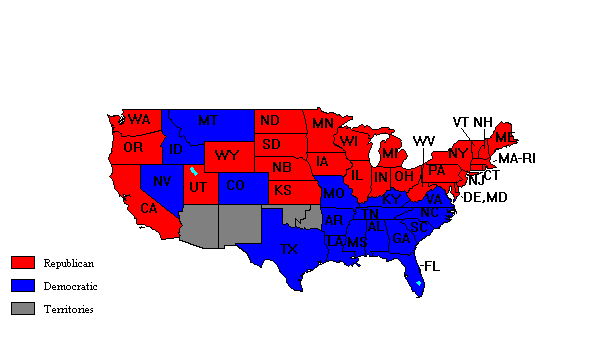Electoral Vote:

Republican (William McKinley) = 292.
Democratic (William Jennings Bryan) = 155.
Total = 447.

Popular Vote:

Republican (William McKinley) = 7,218,039 (51.7%).
Democratic (William Jennings Bryan) = 6,358,345 (45.5%).
Prohibition (John Woolley) = 209,004 (1.5%).
Socialist (Eugene V. Debs) = 86,935 (0.6%).
Minor candidates = 98,147 (0.7%).
Total = 13,970,470.

## 1904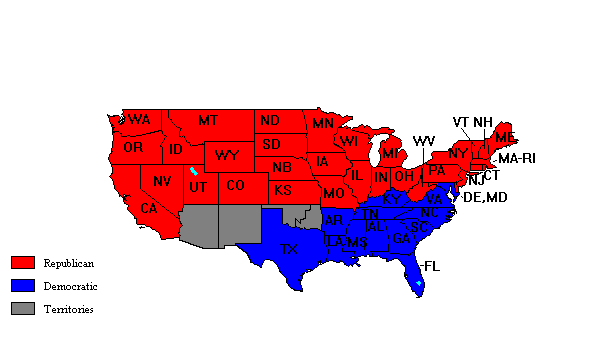Electoral Vote:

Republican (Theodore Roosevelt) = 336.
Democratic (Alton B. Parker) = 140.
Total = 476.

Popular Vote:

Republican (Theodore Roosevelt) = 7,626,593 (56.4%).
Democratic (Alton B. Parker) = 5,082,898 (37.6%).
Socialist (Eugene V. Debs) = 402,489 (3%).
Prohibition (Silas Swallow) = 258,596 (1.9%).
Populist (Thomas Watson) = 114,051 (0.8%).
Minor candidates = 34,337 (0.3%).
Total = 13,518,964.

## 1908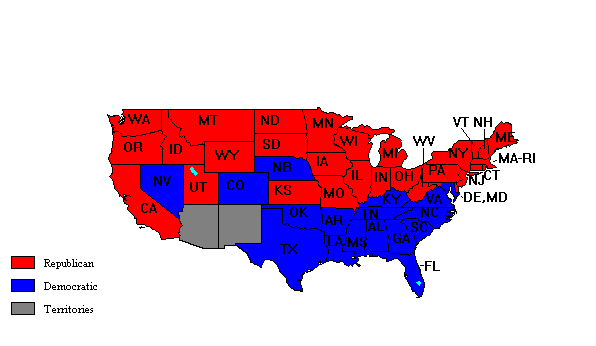Electoral Vote:

Republican (William Howard Taft) = 321.
Democratic (William Jennings Bryan) = 162.
Total = 483.

Popular Vote:

Republican (William Howard Taft) = 7,676,258 (51.6%).
Democratic (William Jennings Bryan) = 6,406,801 (43%).
Socialist (Eugene V. Debs) = 420,380 (2.8%).
Prohibition (Eugene Chafin) = 252,821 (1.7%).
Minor candidates = 126,474 (0.3%).
Total = 14,882,734.

## 1912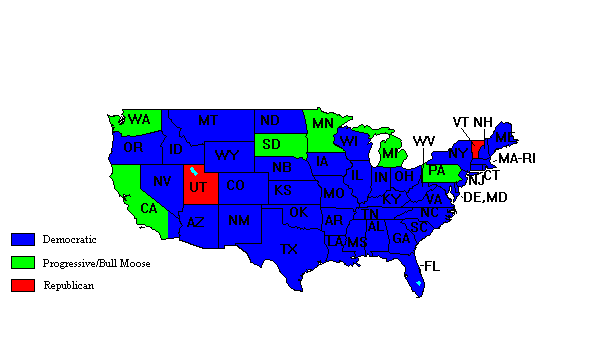Electoral Vote:

Democratic (Woodrow Wilson) = 435.
Progressive/Bull Moose (Theodore Roosevelt) = 88.
Republican (William Howard Taft) = 8.
Total = 531.

Popular Vote:

Democratic (Woodrow Wilson) = 6,293,152 (41.8%).
Progressive/Bull Moose (Theodore Roosevelt) = 4,119,207 (27.4%).
Republican (William Howard Taft) = 3,486,333 (23.2%).
Socialist (Eugene V. Debs) = 900,369 (6%).
Prohibition (Eugene Chafin) = 207,972 (1.4%).
Minor candidates = 33,930 (0.2%).
Total = 15,040,963.

## 1916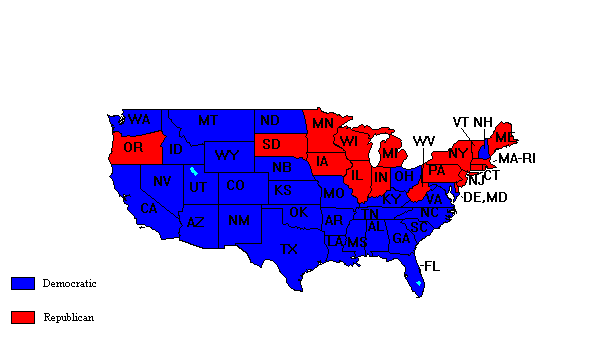Electoral Vote:

Democratic (Woodrow Wilson) = 277.
Republican (Charles E. Hughes) = 254.
Total = 531.

Popular Vote:

Democratic (Woodrow Wilson) = 9,126,300 (49.2%).
Republican (Charles E. Hughes) = 8,546,789 (46.1%).
Socialist (Allan Benson) = 589,924 (3.2%).
Prohibition (James Hanly) = 221,038 (1.2%).
Minor candidates = 50,979 (0.3%).
Total = 18,535,030.

## 1920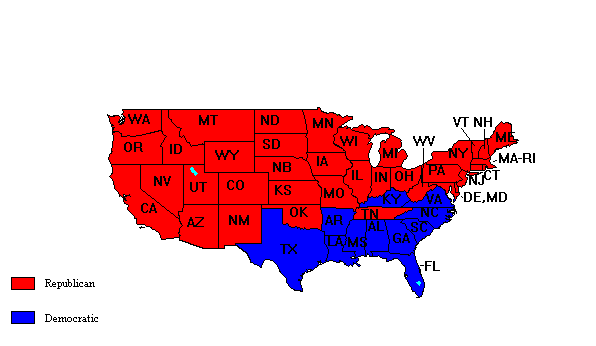Electoral Vote:

Republican (Warren G. Harding) = 404.
Democratic (James Cox) = 127.
Total = 531.

Popular Vote:

Republican (Warren G. Harding) = 16,153,115 (60.3%).
Democratic (James Cox) = 9,133,092 (34.1%).
Socialist (Eugene V. Debs) = 915,490 (3.4%).
Minor candidates = 566,916 (2.2%).
Total = 26,768,613.

## 1924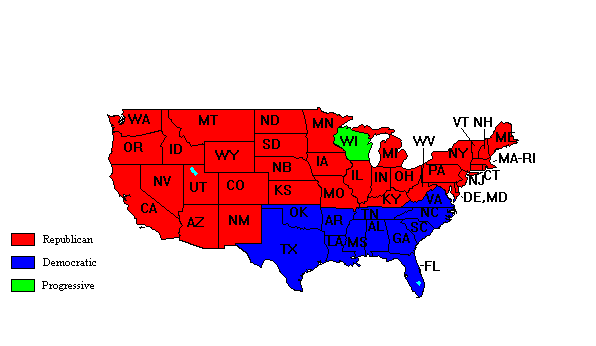Electoral Vote:

Republican (Calvin Coolidge) = 382.
Democratic (John Davis) = 136.
Progressive (Robert LaFollette) = 13.
Total = 531.

Popular Vote:

Republican (Calvin Coolidge) = 15,719,921 (54%).
Democratic (John Davis) = 8,386,704 (28.8%).
Progressive (Robert LaFollette) = 4,832,532 (16.6%).
Minor candidates = 155,866 (0.6%).
Total = 29,095,023.

## 1928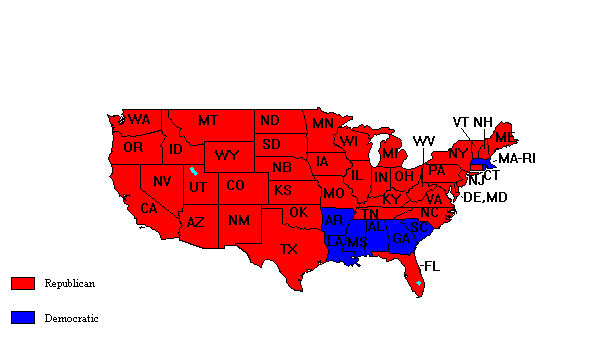Electoral Vote:

Republican (Herbert Hoover) = 444.
Democratic (Alfred E. Smith) = 87.
Total = 531.

Popular Vote:

Republican (Herbert Hoover) = 21,437,277 (58.2%).
Democratic (Alfred E. Smith) = 15,007,698 (40.8%).
Socialist (Norman Thomas) = 265,583 (0.7%).
Minor candidates = 95,393 (0.3%).
Total = 36,805,951.

## 1932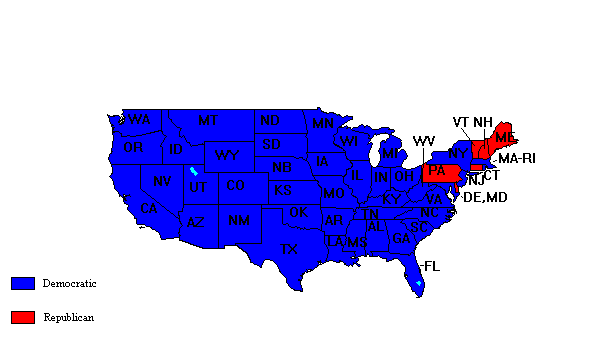Electoral Vote:

Democratic (Franklin Delano Roosevelt) = 472.
Republican (Herbert Hoover) = 59.
Total = 531.

Popular Vote:

Democratic (Franklin Delano Roosevelt) = 22,829,501 (57.42%).
Republican (Herbert Hoover) = 15,760,684 (39.64%).
Socialist (Norman Thomas) = 884,649 (2.23%).
Minor candidates = 283,925 (0.71%).
Total = 39,758,759.

## 1936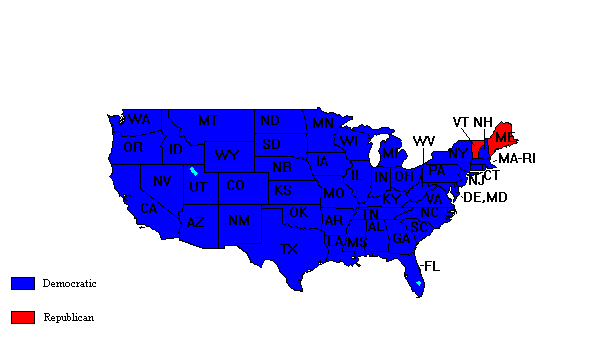Electoral Vote:

Democratic (Franklin Delano Roosevelt) = 523.
Republican (Alfred M. Landon) = 8.
Total = 531.

Popular Vote:

Democratic (Franklin Delano Roosevelt) = 27,757,333 (60.8%).
Republican (Alfred M. Landon) = 16,684,231 (36.5%).
Union (William Lemke) = 892,267 (2%).
Socialist (Norman Thomas) = 187,833 (0.4%).
Minor candidates = 133,099 (0.3%).
Total = 45,654,763.

## 1940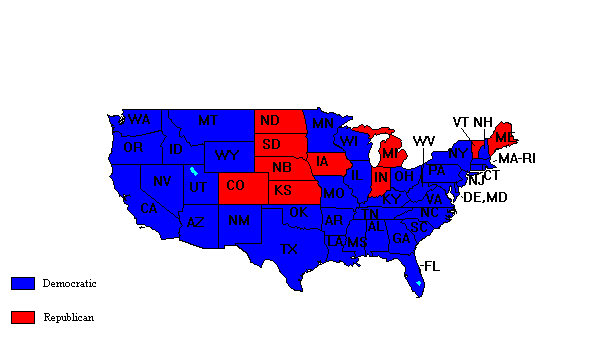Electoral Vote:

Democratic (Franklin Delano Roosevelt) = 449.
Republican (Wendell Willkie) = 82.
Total = 531.

Popular Vote:

Democratic (Franklin Delano Roosevelt) = 27,313,041 (54.7%).
Republican (Wendell Willkie) = 22,348,480 (44.8%).
Minor candidates = 238,897 (0.5%).
Total = 49,900,418.

## 1944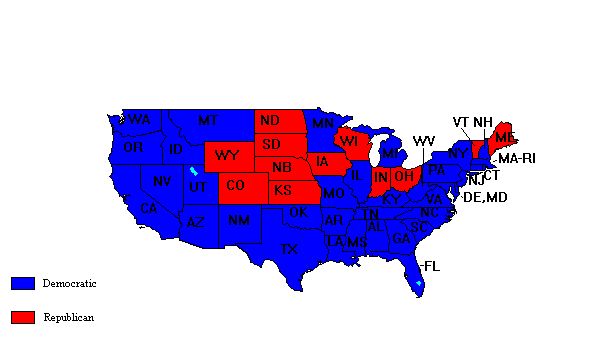Electoral Vote:

Democratic (Franklin Delano Roosevelt) = 432.
Republican (Thomas Dewey) = 99.
Total = 531.

Popular Vote:

Democratic (Franklin Delano Roosevelt) = 25,612,610 (53.4%).
Republican (Thomas Dewey) = 22,017,617 (45.9%).
Minor candidates = 346,463 (0.7%).
Total = 47,976,690.

## 1948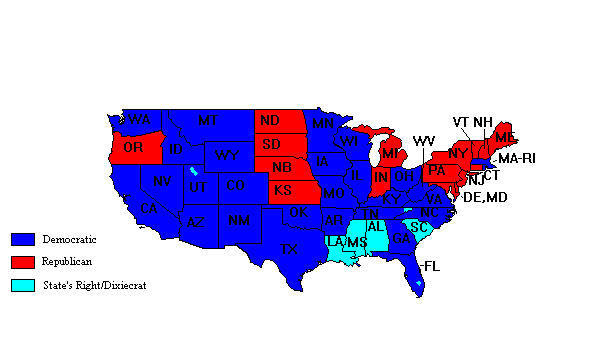Electoral Vote:

Democratic (Harry Truman) = 303.
Republican (William Howard Taft) = 189.
State's Right/Dixiecrat (Strom Thurmond) = 39.
Total = 531.

Popular Vote:

Democratic (Harry Truman) = 24,179,345 (49.6%).
Republican (Thomas Dewey) = 21,991,291 (45%).
State's Right/Dixiecrat (Strom Thurmond) = 1,176,125 (2.4%).
Progressive (Henry Wallace) = 1,157,326 (2.4%).
Minor candidates = 289,739 (0.6%).
Total = 48,793,826.

## 1952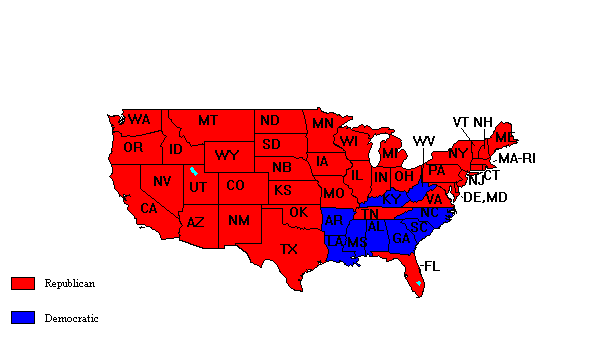Electoral Vote:

Republican (Dwight Eisenhower) = 442.
Democratic (Adlai Stevenson) = 89.
Total = 531.

Popular Vote:

Republican (Dwight Eisenhower) = 33,936,234 (55.1%).
Democratic (Adlai Stevenson) = 27,314,992 (44.4%).
Minor candidates = 299,692 (0.5%).
Total = 61,550,918.

## 1956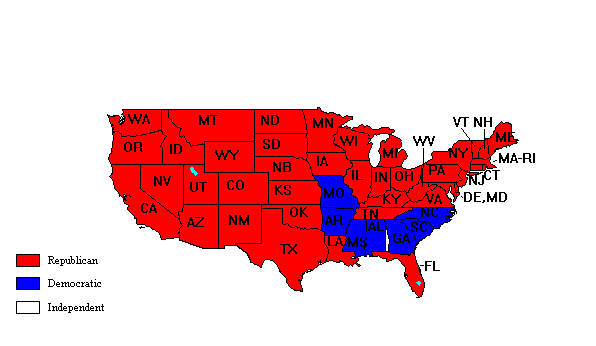Electoral Vote:

Republican (Dwight Eisenhower) = 457.
Democratic (Adlai Stevenson) = 73.
Independent (Walter Jones) = 1.
Total = 531.

Popular Vote:

Republican (Dwight Eisenhower) = 35,590,472 (57.4%).
Democratic (Adlai Stevenson) = 26,022,752 (42%).
Minor candidates = 413,684 (0.6%).
Total = 62,026,908.

## 1960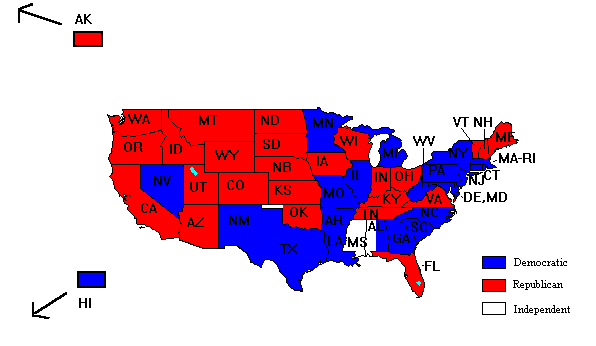Electoral Vote:

Democratic (John F. Kennedy) = 303.
Republican (Richard M. Nixon) = 219.
Independent (Harry Byrd) = 15.
Total = 537.

Popular Vote:

Democratic (John F. Kennedy) = 34,226,731 (49.7%).
Republican (Richard M. Nixon) = 34,108,157 (49.6%).
Minor candidates = 503,331 (0.7%).
Total = 68,838,219.

## 1964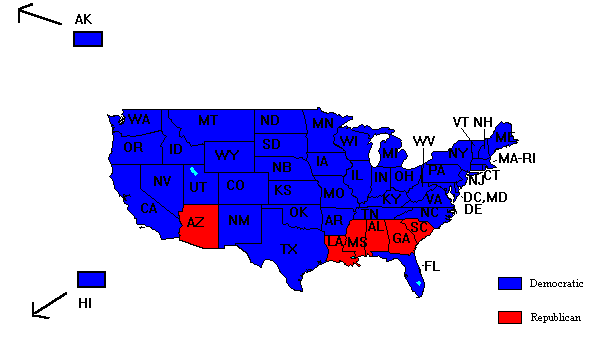Electoral Vote:

Democratic (Lyndon Johnson) = 486.
Republican (Barry Goldwater) = 52.
Total = 538.

Popular Vote:

Democratic (Lyndon Johnson) = 43,129,566 (61.05%).
Republican (Barry Goldwater) = 27,178,188 (38.47%).
Minor candidates = 336,838 (0.48%).
Total = 70,644,592.

## 1968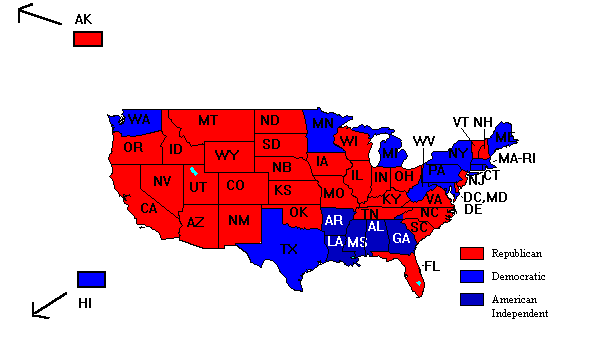Electoral Vote:

Republican (Richard M. Nixon) = 301.
Democratic (Hubert Humphrey) = 191.
American Independent (George Wallace) = 46.
Total = 538.

Popular Vote:

Republican (Richard M. Nixon) = 31,785,480 (43.42%).
Democratic (Hubert Humphrey) = 31,275,166 (42.72%).
American Independent (George Wallace) = 9,906,473 (13.53%).
Minor candidates = 244,756 (0.33%).
Total = 73,211,875.

## 1972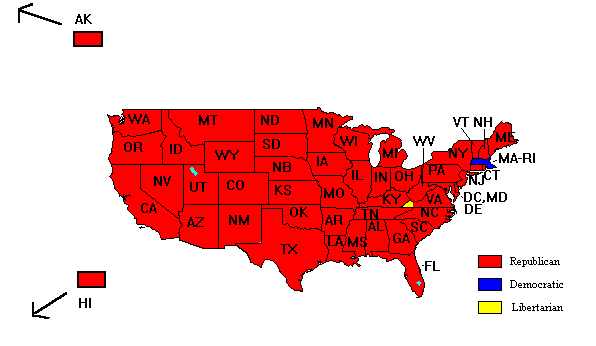Electoral Vote:

Republican (Richard M. Nixon) = 520.
Democratic (George McGovern) = 17.
Libertarian (John Hospers) = 1.
Total = 538.

Popular Vote:

Republican (Richard M. Nixon) = 47,169,911 (60.7%).
Democratic (George McGovern) = 29,170,383 (37.5%).
American Independent (John Schmitz) = 1,099,482 (1.4%).
Minor candidates = 278,778 (0.4%).
Total = 77,718,554.

## 1976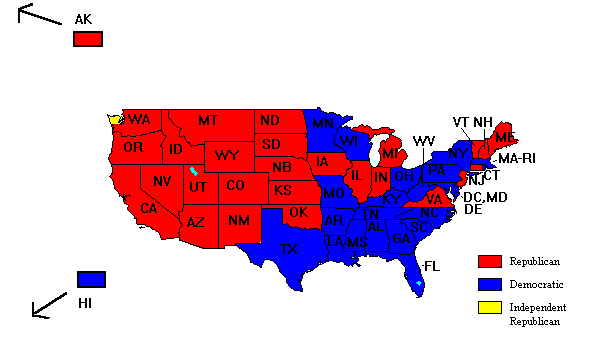Electoral Vote:

Democratic (Jimmy Carter) = 297.
Republican (Gerald Ford) = 239.
Independent Republican (Ronald Reagan) = 1.
Total = 538.

Popular Vote:

Democratic (Jimmy Carter) = 40,830,763 (50.1%).
Republican (Gerald Ford) = 39,147,793 (48%).
Independent (Eugene McCarthy) = 756,691 (0.9%).
Minor candidates = 820,642 (1%).
Total = 81,555,889.

## 1980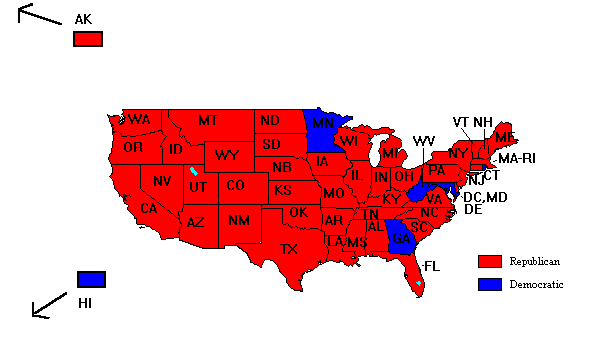Electoral Vote:

Republican (Ronald Reagan) = 489.
Democratic (Jimmy Carter) = 49.
Total = 538.

Popular Vote:

Republican (Ronald Reagan) = 43,904,153 (50.7%).
Democratic (Jimmy Carter) = 35,483,883 (41%).
Independent (John Anderson) = 5,720,060 (6.6%).
Libertarian (Ed Clark) = 921,299 (1.1%).
Minor candidates = 485,826 (0.6%).
Total = 86,515,221.

## 1984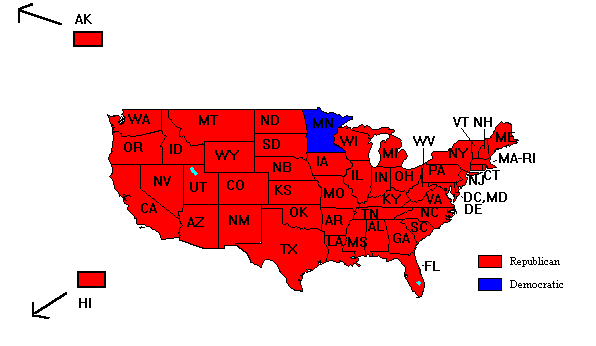Electoral Vote:

Republican (Ronald Reagan) = 525.
Democratic (Walter Mondale) = 13.
Total = 538.

Popular Vote:

Republican (Ronald Reagan) = 54,455,075 (58.8%).
Democratic (Walter Mondale) = 37,577,185 (40.6%).
Libertarian (David Bergland) = 228,314 (0.2%).
Minor candidates = 392,268 (0.4%).
Total = 92,652,842.

## 1988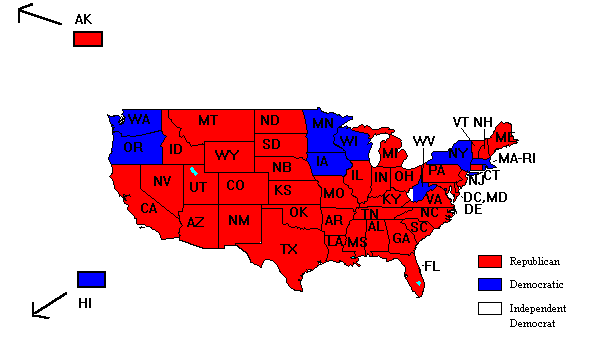Electoral Vote:

Republican (George H. Bush) = 426.
Democratic (Michael Dukakis) = 111.
Independent Democrat (Lloyd Bentsen) = 1.
Total = 538.

Popular Vote:

Republican (George H. Bush) = 48,886,588 (53.37%).
Democratic (Michael Dukakis) = 41,809,485 (45.65%).
Libertarian (Ron Paul) = 432,184 (0.47%).
Minor candidates = 467,463 (0.51%).
Total = 91,595,720.

## 1992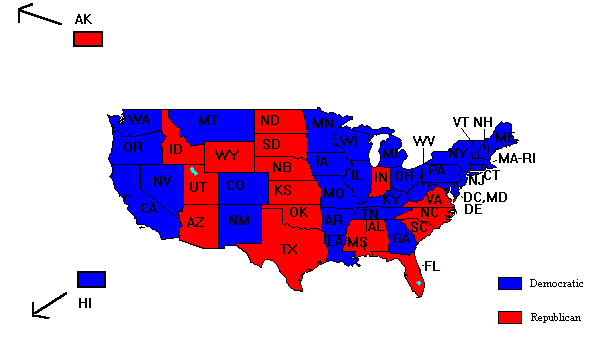Electoral Vote:

Democratic (Bill Clinton) = 370.
Republican (George H. Bush) = 168.
Total = 538.

Popular Vote:

Democratic (Bill Clinton) = 44,909,806 (43.01%).
Republican (George H. Bush) = 39,104,550 (37.45%).
Reform (H. Ross Perot) = 19,742,240 (18.91%).
Libertarian (Andre Marrau) = 291,631 (0.28%).
Minor candidates = 378,531 (0.36%).
Total = 104,426,758.

## 1996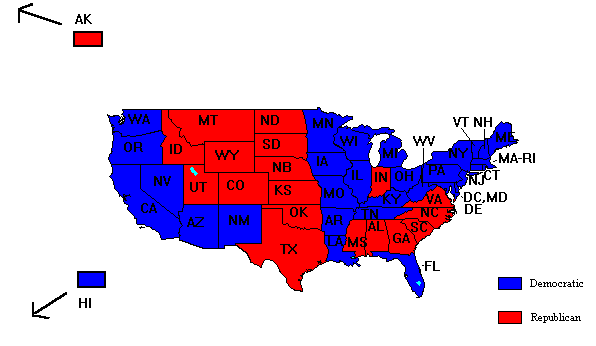Electoral Vote:

Democratic (Bill Clinton) = 379.
Republican (Bob Dole) = 159.
Total = 538.

Popular Vote:

Democratic (Bill Clinton) = 47,402,357 (49.24%).
Republican (Bob Dole) = 39,198,755 (40.71%).
Reform (H. Ross Perot) = 8,085,402 (8.4%).
Green (Ralph Nader) = 685,128 (0.71%).
Libertarian (Harry Browne) = 485,798 (0.5%).
Minor candidates = 420,194 (0.44%).
Total = 96,277,634.

## 2000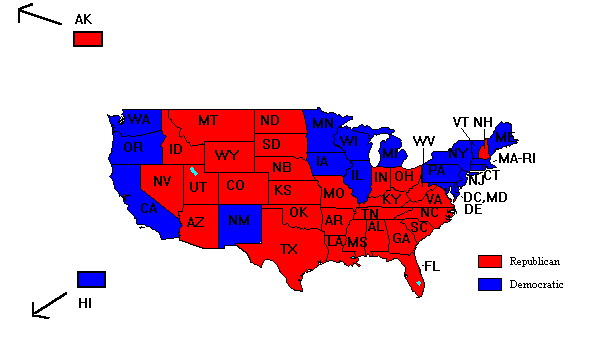Electoral Vote:

Republican (George W. Bush) = 271.
Democratic (Albert Gore) = 266.
Not voting = 1.
Total = 538.

Popular Vote:

Democratic (Albert Gore) = 51,003,238 (48.38%).
Republican (George W. Bush) = 50,459,624 (47.87%).
Green (Ralph Nader) = 2,882,985 (2.74%).
Reform (Patrick Buchanan) = 449,120 (0.43%).
Libertarian (Harry Browne) = 384,440 (0.36%).
Minor candidates = 232,922 (0.22%).
Total = 105,412,329.

## 2004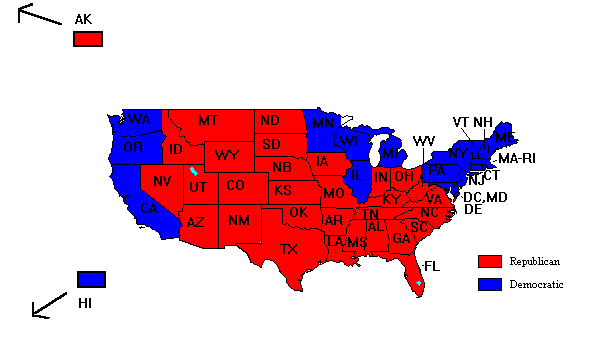Electoral Vote:

Republican (George W. Bush) = 286.
Democratic (John F. Kerry) = 251.
Independent Democrat (John Edwards) = 1.
Total = 538.

Popular Vote:

Republican (George W. Bush) = 61,981,080 (50.73%).
Democratic (John F. Kerry) = 58,962,408 (48.26%).
Independent (Ralph Nader) = 460,189 (0.38%).
Libertarian (Michael Badnarik) = 396,526 (0.32%).
Minor candidates = 367,205 (0.30%).
Total = 122,167,408.

## 2008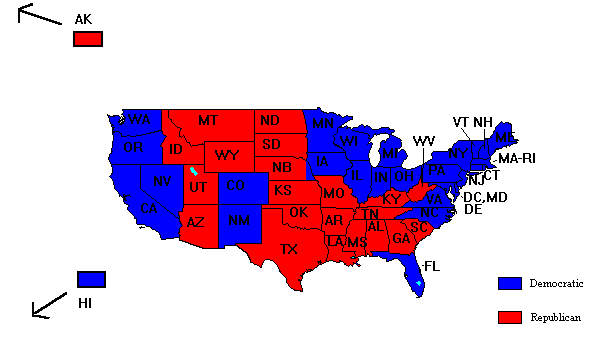Electoral Vote:

Democratic (Barack Obama) = 365.
Republican (John McCain) = 173.
Total = 538.

Popular Vote:

Democratic (Barack Obama) = 69,456,898 (52.87%).
Republican (John McCain) = 59,934,814 (45.62%).
Independent (Ralph Nader) = 738,771 (0.56%).
Libertarian (Bob Barr) = 523,686 (0.40%).
Minor candidates = 716,624 (0.55%).
Total = 131,370,793.

## 2012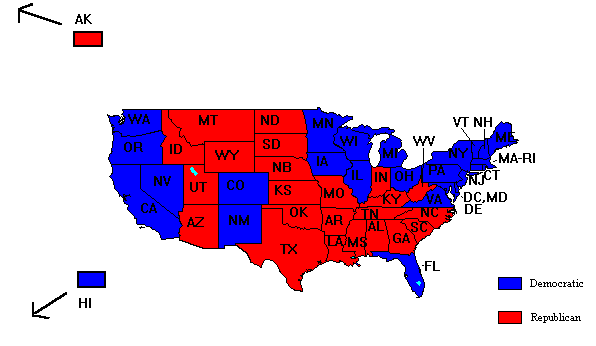Electoral Vote:

Democratic (Barack Obama) = 332.
Republican (Mitt Romney) = 206.
Total = 538.

Popular Vote:

Democratic (Barack Obama) = 65,918,507 (51.01%).
Republican (Mitt Romney) = 60,934,407 (47.15%).
Libertarian (Gary Johnson) = 1,275,923 (0.99%).
Green (Jill Stein) = 469,015 (0.36%).
Minor candidates = 639.790 (0.50%).
Total = 129,237,642.

## 2016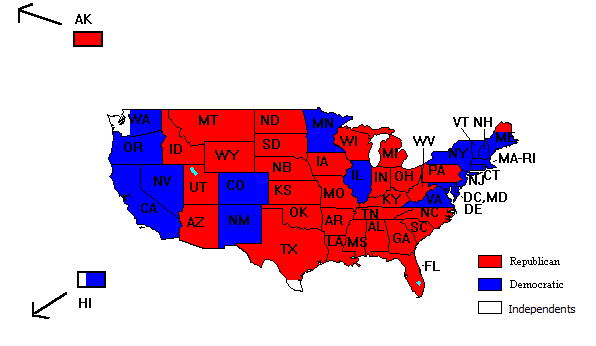Electoral Vote:

Republican (Donald Trump) = 304.
Democratic (Hillary Clinton Obama) = 227.
Independent (Colin Powell, cast in WA) = 3
Independent (Faith Spotted Eagle, cast in WA) = 1
Independent Democrat (Bernie Sanders, cast in HI) = 1
Independent Republican (John Kasich, cast in TX) = 1
Independent Republican (Ron Paul, cast in TX) = 1
Total = 538.

Popular Vote:

Democratic (Hillary Clinton) = 65,853,652 (48.02%).
Republican (Donald Trump) = 62,985,134 (45.93%).
Libertarian (Gary Johnson) = 4,489,235 (3.27%).
Green (Jill Stein) = 1,457,226 (1.06%).
Independent (Evan McMullin) = 732,273 (0.53%).
Minor candidates = 1,607,964 (1.17%).
Total = 137,125,484.

The data for this page came from the following sources:

Support this site!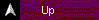# PAGE  NAVIGATOR

### Beyond HistoryVisitors: